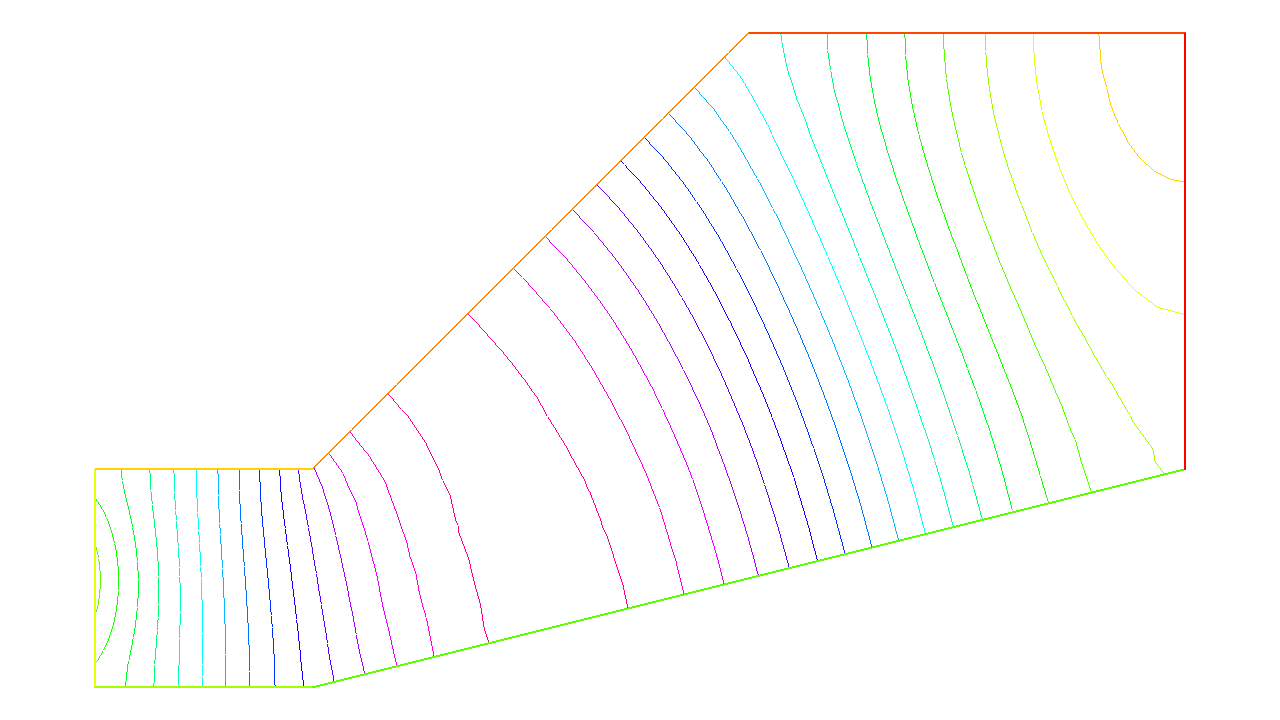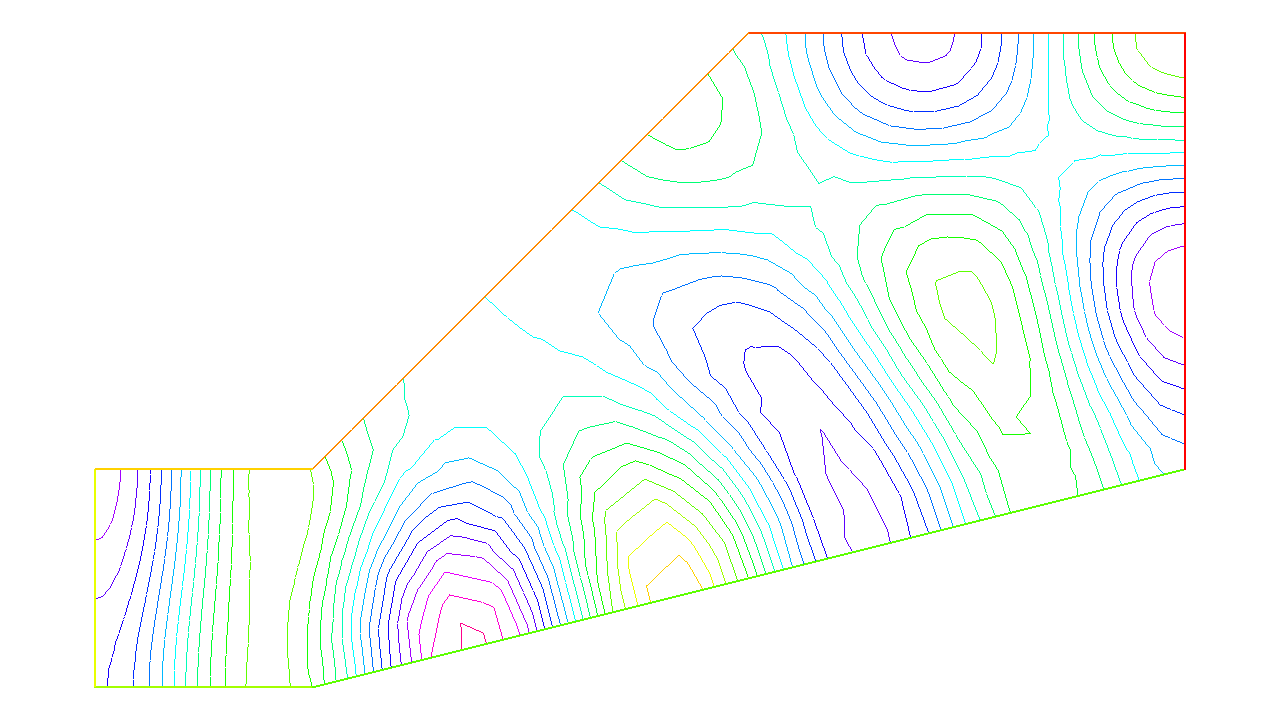FreeFEM Documentation on GitHub

stars - forks

# Acoustics

Summary : Here we go to grip with ill posed problems and eigenvalue problems

Pressure variations in air at rest are governed by the wave equation:

${\partial^2 u \over \partial t^2} - c^2 \Delta u =0$

When the solution wave is monochromatic (and that depends on the boundary and initial conditions), $$u$$ is of the form $$u(x,t)=Re(v(x) e^{ik t})$$ where $$v$$ is a solution of Helmholtz’s equation:

$\begin{split}\begin{array}{rcl} k^{2}v + c^{2}\Delta v &= 0 &\hbox{ in } \Omega\\ \frac{\partial v}{\partial\boldsymbol{n}}|_\Gamma &= g & \end{array}\end{split}$

where $$g$$ is the source.

Note the “+” sign in front of the Laplace operator and that $$k > 0$$ is real. This sign may make the problem ill posed for some values of $$\frac c k$$, a phenomenon called “resonance”.

At resonance there are non-zero solutions even when $$g=0$$. So the following program may or may not work:

 1// Parameters
2real kc2 = 1.;
3func g = y*(1.-y);
4
5// Mesh
6border a0(t=0., 1.){x=5.; y=1.+2.*t;}
7border a1(t=0., 1.){x=5.-2.*t; y=3.;}
8border a2(t=0., 1.){x=3.-2.*t; y=3.-2.*t;}
9border a3(t=0., 1.){x=1.-t; y=1.;}
10border a4(t=0., 1.){x=0.; y=1.-t;}
11border a5(t=0., 1.){x=t; y=0.;}
12border a6(t=0., 1.){x=1.+4.*t; y=t;}
13
14mesh Th = buildmesh(a0(20) + a1(20) + a2(20)
15    + a3(20) + a4(20) + a5(20) + a6(20));
16
17// Fespace
18fespace Vh(Th, P1);
19Vh u, v;
20
21// Solve
22solve sound(u, v)
23   = int2d(Th)(
24        u*v * kc2
25      - dx(u)*dx(v)
26      - dy(u)*dy(v)
27   )
28   - int1d(Th, a4)(
29        g * v
30   )
31   ;
32
33// Plot
34plot(u, wait=1, ps="Sound.eps");


Results are on Fig. 11. But when $$kc2$$ is an eigenvalue of the problem, then the solution is not unique:

• if $$u_e \neq 0$$ is an eigen state, then for any given solution $$u+u_e$$ is another solution.

To find all the $$u_e$$ one can do the following :

 1// Parameters
2real sigma = 20; //value of the shift
3
4// Problem
5// OP = A - sigma B ; // The shifted matrix
6varf op(u1, u2)
7   = int2d(Th)(
8        dx(u1)*dx(u2)
9      + dy(u1)*dy(u2)
10      - sigma* u1*u2
11   )
12   ;
13
14varf b([u1], [u2])
15   = int2d(Th)(
16        u1*u2
17   )
18   ; // No Boundary condition see note \ref{note BC EV}
19
20matrix OP = op(Vh, Vh, solver=Crout, factorize=1);
21matrix B = b(Vh, Vh, solver=CG, eps=1e-20);
22
23// Eigen values
24int nev=2; // Number of requested eigenvalues near sigma
25
26real[int] ev(nev);  // To store the nev eigenvalue
27Vh[int] eV(nev);    // To store the nev eigenvector
28
29int k=EigenValue(OP, B, sym=true, sigma=sigma, value=ev, vector=eV,
30   tol=1e-10, maxit=0, ncv=0);
31
32cout << ev(0) << " 2 eigen values " << ev(1) << endl;
33v = eV;
34plot(v, wait=true, ps="eigen.eps");Fig. 11 Amplitude of an acoustic signal coming from the left vertical wall.Fig. 12 First eigen state ($$\lambda=(k/c)^2=14.695$$) close to $$15$$ of eigenvalue problem: $$-\Delta \varphi = \lambda\varphi$$ and $$\frac{\partial \varphi}{\partial\boldsymbol{n}} = 0$$ on $$\Gamma$$}

Acoustics

Table of content